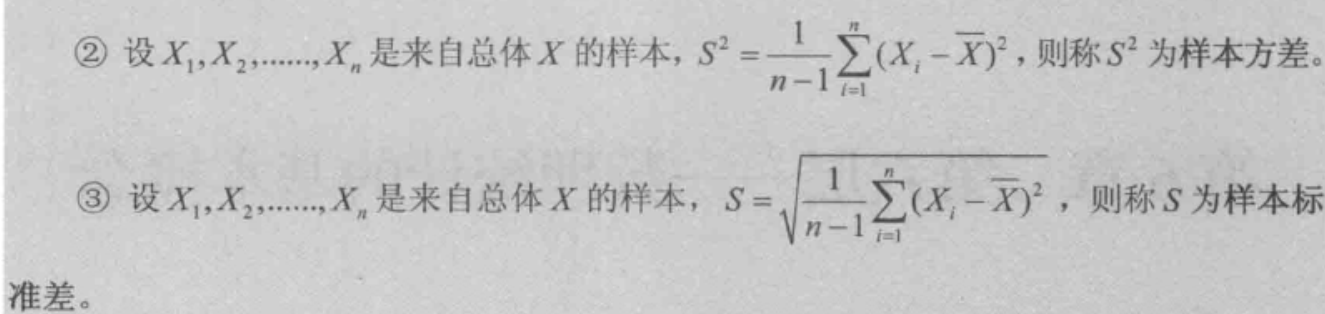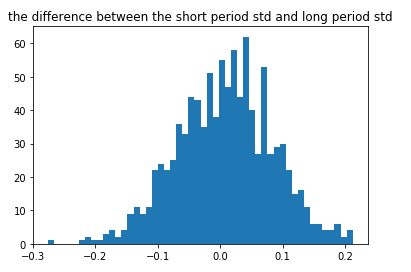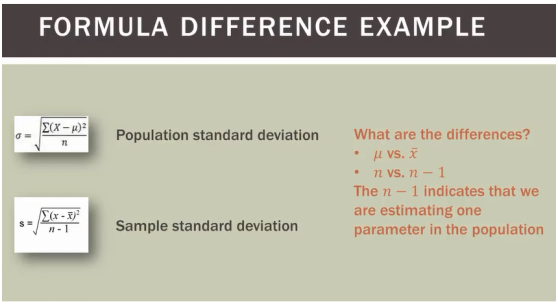•展开全文• import pandas as pd import numpy as np ...如上，通过模拟生成10万个标准正态分布的随机数，然后分别随机抽样100个和总体的计算标准差的差值，发现差值的期望为0，也就是可以认为这种状态下的标准差是无偏估计？
import pandas as pd
import numpy as np
import matplotlib.pyplot as plt
rate_list=np.random.randn(100000)
result=[]
for i in range(1000):
rate_short=np.random.choice(rate_list,100)
rate_long=0rate_list[:]
result.append(rate_long.std()-rate_short.std())
result=pd.Series(result)
plt.hist(result,bins=50)
plt.title('the difference between the short period std and long period std')
plt.show()如上，通过模拟生成10万个标准正态分布的随机数，然后分别随机抽样100个和总体的计算标准差的差值，发现差值的期望为0，也就是可以认为这种状态下的标准差是无偏估计？展开全文• 1，求一组数据的标准差，该组数据就是总体，此时是求总体标准差，公式中分母为n； 2，总体太大或未知，只能每次抽取样本，此时是求样本标准差，分母为n-1。 图片来源：...
总体标准差和样本标准差公式：解释：

1，求一组数据的标准差，该组数据就是总体，此时是求总体标准差，公式中分母为n；

2，总体太大或未知，只能每次抽取样本，此时是求样本标准差，分母为n-1。

图片来源：https://www.cnblogs.com/webRobot/p/7722820.html

展开全文• 其中 sss 为样本的标准差。该式子的含义是 (n-1)*样本方差与总体方差之比服从自由度为 n-1 的卡方分布。 一直以为这个式子很好证，自己试着没证出来。最后查了查，其实并不好证，要利用到不熟悉的高...
在一本统计学课本中见到一个熟悉的式子：
$\frac{(n-1)s^2}{\sigma^2}\sim \chi^2(n-1)$
其中 $s$ 为样本的标准差。该式子的含义是 (n-1)*样本方差与总体方差之比服从自由度为 n-1 的卡方分布。而且，$\overline{X}$ 与 $S^2$ 相互独立。
一直以为这个式子很好证，自己试着没证出来。最后查了查，其实并不好证，要利用到不熟悉的高深性质。
证明：
\begin{aligned} \frac{(n-1)s^2}{\sigma^2}=&\frac{\sum_{i=1}^n (x_i-\overline{x})^2}{\sigma^2}\\ =&\sum_{i=1}^n (\frac{x_i}{\sigma}-\frac{\overline{x}}{\sigma})^2 \\ =&\sum_{i=1}^n (\frac{x_i-\mu}{\sigma}-\frac{\overline{x}-\mu}{\sigma})^2 \\ =&\sum_{i=1}^n (Z_i-\overline{Z})^2 \\ =& \sum_{i=1}^n Z_i ^2 -n\overline{Z}^2 \end{aligned}
其中 $Z_i$ 表示一个标准正态分布变量。下面利用 “一组相互独立的标准正态随机变量经过正交变换后仍然是相互独立的标准正态随机变量”的性质。
构造一个正交矩阵 $A$，对 $Z_i$ 做正交变换，即 $Y=AZ$，其中 $Z=(Z_1, Z_2,\dots, Z_n)^T$，$Y=(Y_1, Y_2, \dots, Y_n)^T$.
$\sum Y_i^2=Y^TY=Z^TA^TAZ=Z^TZ=\sum Z_i^2$
令 $A_1=(\frac{1}{\sqrt{n}},\frac{1}{\sqrt{n}}\dots,\frac{1}{\sqrt{n}})$，（其他 $A_i$ 的值不用管，只要满足正交矩阵即可），则
$n\overline{Z}^2=\frac{(Z_1+Z_2+\dots+Z_n)^2}{n}=(A_1Z)^2=Y_1^2$
所以：
\begin{aligned} \frac{(n-1)s^2}{\sigma^2}=& \sum_{i=1}^n Z_i ^2 -n\overline{Z}^2 \\ =&\sum Y_i^2-Y_1^2=\sum_{i=2}^n Y_i^2=\chi^2(n-1） \end{aligned}
只要该性质成立，则上面结论就成立了。下面证明这个性质成立，设 $a_{i,j}$ 为正交矩阵 $A$ 中的元素，
$E(Y_i)=\sum_{j=1}^n a_{ij} Z_j=0$
$E(Y_i^2)=E(\sum_{j=1}^n a_{ij} Z_j)^2=a_{i,1}^2E(Z_1^2)+\dots+a_{i,n}^2E(Z_n^2)=a_{i,1}^2+\dots+a_{i,n}^2=1$
$i$ 不等于 $j$ 时，
\begin{aligned} Cov(Y_i, Y_j)=&E(Y_i Y_j)-E(Y_i)E(Y_j) \\ =&E(Y_i Y_j) \\ =&\sum_{k=1}^{n} a_{i,k}^2a_{j,k}^2=0 \end{aligned}
因此可以得出 $\sum_{i=2}^n Y_i^2=\chi^2(n-1)$ 成立，上面利用了正交矩阵的性质，因此性质 “一组相互独立的标准正态随机变量经过正交变换后仍然是相互独立的标准正态随机变量” 成立，原结论也成立。
$\square$
其实利用一个定理 Cochran’s theorem，可以推出
$\sum_{i=1}^n (x_i-\overline{x})^2\sim \sigma^2\chi^2(n-1)$
就可以一步得出了，不过这个定理不太好理解。
利用这个性质，就可以做 t 检验了，因为：
$\frac{\overline{X}-\mu}{S/\sqrt{n}}\sim t(n-1)$
（2）对于相互独立性，由于 $S^2$ 只与 $Y_2, Y_3, \dots, Y_n$ 有关，而 $\frac{\overline{X}-\mu}{\sigma}=\overline{Z}=\frac{1}{\sqrt{n}}Y_1$，可见 $\overline{X}$ 只与 $Y_1$ 有关，由 $Y_1, Y_2, \dots, Y_n$ 的独立性，知道  $\overline{X}$ 与 $S^2$ 相互独立。


展开全文卡方分布
• 方差和标准差是概率与统计学里...由于方差和标准差差一个根号，所以接下来我主要介绍样本方差和母体方差，样本标准差和母体标准差的区别可以依样画葫芦 样本方差和母体方差区别 这里我先举一个例子简单说明...
• 如何理解总体标准差样本标准差与标准误 1 总体标准差 已知随机变量 XXX 数学期望为 μ\muμ，标准差为 σ\sigmaσ，则其方差为： σ2=E[(X−μ)2] \sigma^2=E[(X-\mu)^2] σ2=E[(X−μ)2]此处 σ\sigmaσ 即为...统计学 大数据 机器学习 数据分析
• 也叫无偏样本标准差，就是自由度为 n-1 代码 imimport numpy as np each_acc1 = [1, 2, 3, 4, 5, 6, 7, 8, 9, 10] print("总体标准差:", np.std(each_acc1)) print("样本标准差:", np.std(...
• 标准差 对于前面例子数据 [1,2,5,8,9]，求出来方差是10，但是这个方差...为了保持单位一致性，我们引入一个新统计量--标准差 公式：o=√(o^2) 这样原始数据和标准差的单位就一致了 标准差越大，表示数据越分散
• select STDDEV(b.sectionscore)s,---------------这里是样本标准差不是全部数据... STDDEV_POP(b.sectionscore)s,---------------这个是所有数据的标准差 fromsubjectregisteraleftjoinsubjectsectionscorebona....
• （1）式是总体标准差，（2）式是样本标准差 Std(A,flag)中： flag表示标注公差时是要除以n还是n－1 flag0…是除以n－1，总体标准差 flag1…是除以n，样本标准差 std(A,flag,dim) dim表示维数 dim1…是按照列计算 dim...matlab
• 标准差（Standard Deviation） ，中文环境中又常称均方差，是离均差平方算术平均数平方根，用σ表示。标准差是方差算术平方根。标准差能反映一个数据集离散程度。平均数相同两组数据，标准差未必相同。...
• 假设总体数量为（m+n），其包含两个亚组（，），第一组平均值和标准差分别为和，第二组平均值和标准差分别为和，则总体平均值和标准差是多少呢？ 先给答案： ， 平均值推导过程： 标准差推导过程： ...
• 估计量评选基本标准有 无偏性、有效性、相合性，很难有都满足情况，一般用Bessel纠正方法，作为样本方差对总体方差无偏估计量统计学
• 注：样本方差和样本标准差都是衡量一个样本波动大小量，样本方差或样本标准差越大，样本数据波动就越大。 标准差与标准方差 1、定义：方差是各个数据与平均数之差平方和平均数。在概率论和数理统计中，...
• 为了提高EWMA标准差控制图检测速度,提出变化抽样区间和样本容量EWMA标准差控制图,并同传统EWMA和变化抽样区间EWMA标准差控制图进行比较.最后模拟结果显示对于较小方差漂移,VSSI EWMA标准差控制图检测速度...
• 许多paper里经常能看到Mean±SD（SE）这样表达方式，或者在图表里用SD或者SE来表示error bar，用SD居多，但是也有不少用SE。初学者很容易混淆SD（standard deviation）和SE（standard error）。 SD SD我们都很...
• 如是总体，标准差公式根号内除以n如是样本标准差公式根号内除以(n-1)(样本至少比总体个数少一)因为我们大量接触样本，所以普遍使用根号内除以(n-1) 除以n-1是为了保证样本方差是总体方差一个无偏估计。。...
• 大家好，今天给大家介绍标准差。标准差在统计领域是一个重要...用numpy模块计算，两组数的标准差相差10倍 方差是实际值与期望值之差平方的平均值。方差，通俗点讲，就是和中心偏离的程度!用来衡量一批数据的波动数据分析 python 数学
• 参考：http://www.cnblogs.com/cvlabs/archive/2010/03/26/1696978.html  ... ​ 浙江大学概率论与数理统计 ...方差：方差是变量与其平均值平方和算术平均值，例如：
• 但是在真实世界中，找到一个总体的标准差是不现实的。大多数情况下，我们都是通过计算样本标准差（s）来估计总体标准差（σ）的。但是s的计算公式是这样的： 分母为什么要（n-1）呢，而不是n？ 维基百科给出...
• 样本均值：mean 　M = mean(A) : A可以是向量，返回向量元素的样本均值；...　M = mean(A, dim) : 如果A是一个矩阵，则mean(A,2)返回是一个列向量，列向量中元素值代表每个行向量的样本均值； 标...
• 本文转自二郎三郎博客园博客，原文链接：http://www.cnblogs.com/haore147/p/3640400.html，如需转载请自行联系原作者 ...
• 求它的标准差，我们知道公式是： 分母为什么是n-1而不是n呢？ 这个问题需要我们从统计学的知识开始说起来，统计学就是通过对一部分数据（样本）的观察分析，来获取整体数据的特征。也就是说我们的目的是为了获得...测试
• 许多paper里经常能看到Mean±SD（SE）这样表达方式，或者在图表里用SD或者SE来表示error bar，用SD居多，但是也有不少用SE。初学者很容易混淆SD（standard deviation）和SE（standard error）。SDSD我们都很...
• 在只有均值、标准差样本情况下，spss是不能实现方差分析。因此，这个小小软件能够帮助你问题。spss 方差分析...